# 1. VAT Exchange Rate Amount

In the following section we discuss how to specify and treat the exchange rate for VAT items that differ from those in the general ledger and how to deal with these difference.

Exchange rates determined in accordance with the Accounting Act and the VAT Act may differ. Use the function below to handle these differences.

The VAT base and the VAT amount are calculated using the exchange rate specified on the VAT completion date. Receivables and liabilities in the buyer and supplier items are calculated using the exchange rates prevailing at the accounting date. Ledger items are also calculated at the exchange rate as of the Posting Date except for items that include VAT which are calculated at the VAT rate. In order for the ledger items and the VAT items to be the same an adjustment item must be posted to the ledger items with the difference calculated at the exchange rate difference.

Example:

VAT rate management is available in several locations. In this example we show you how to use it through a Sales Invoice. Based on the initial data the VAT Date and the Posting Date are the same and can be checked in the general tab of the order.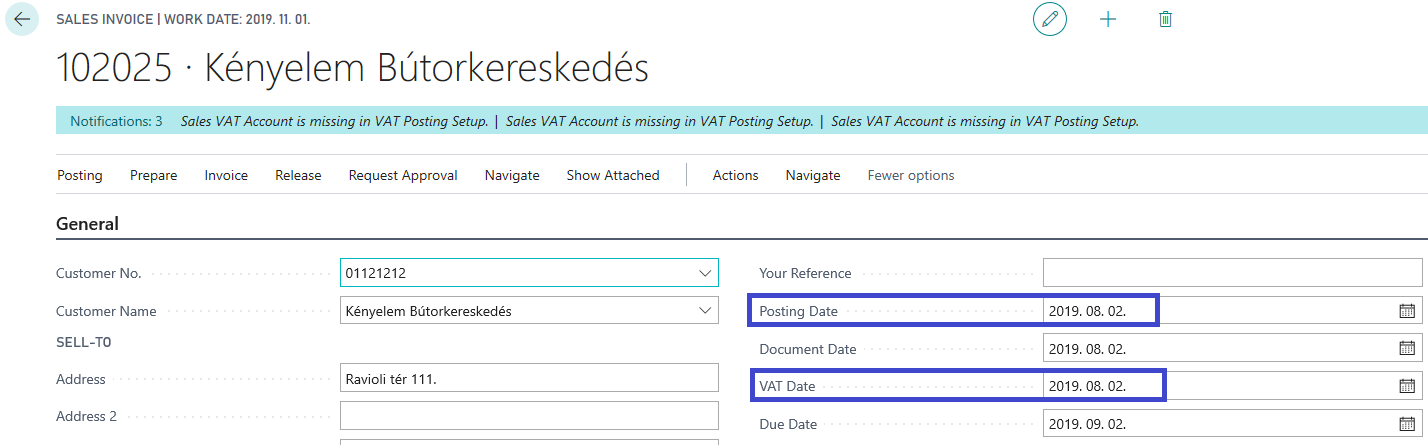By clicking on the three dots next to the Currency Code field on the Invoice Details page you can see that the Relational Exch. Amount Rate Amount and the VAT Exchange Rate Amount are the same.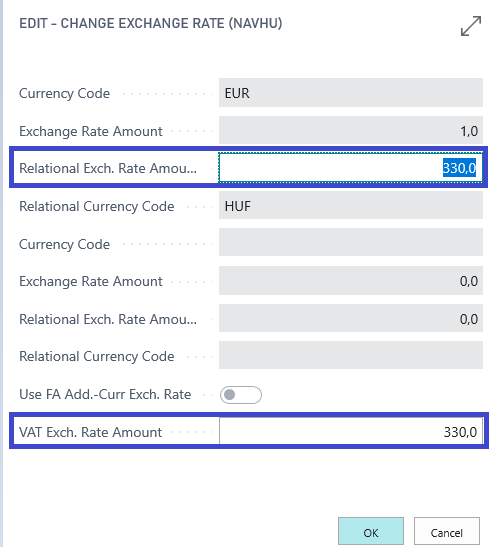The next step is to adjust the two dates so that they don’t match.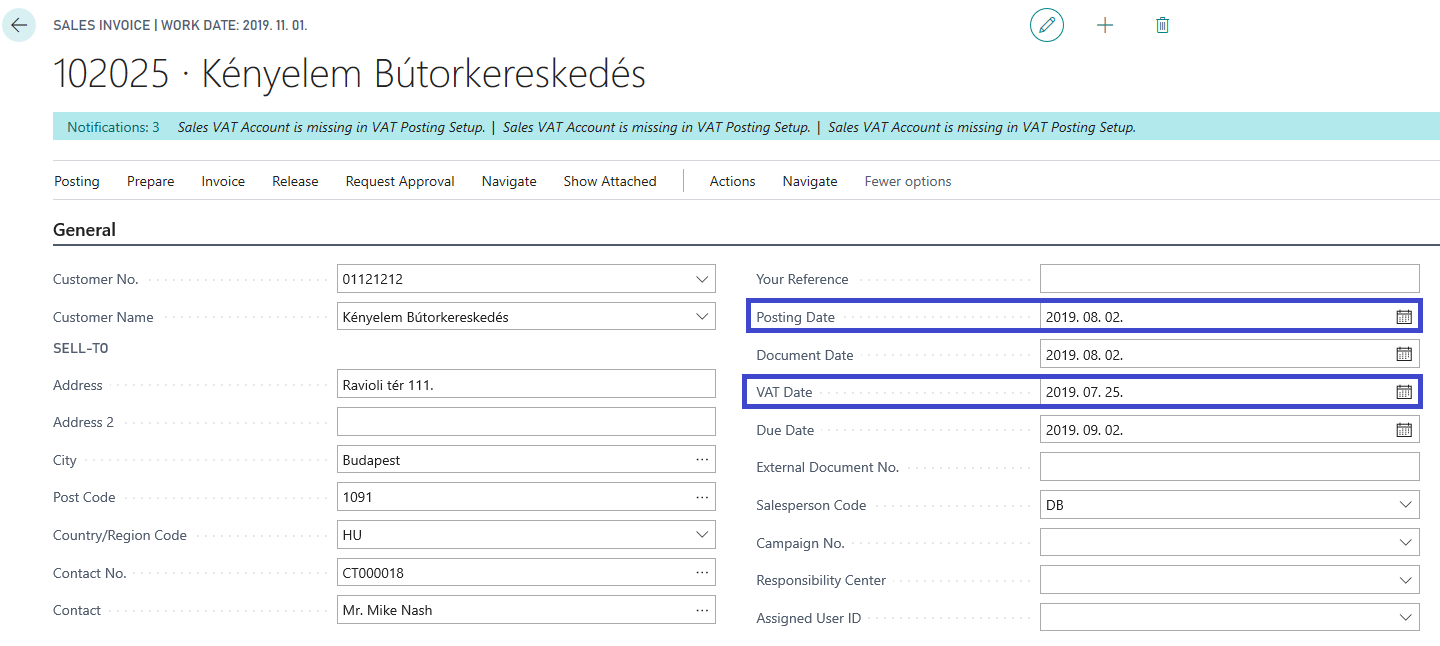After we changed the VAT Date the VAT Exch. Rate Amount changed also.The exchange rates are derived from the Currencies page.

# 2. VAT Posting Setup

For this function to work, you need to enter the Sales price in the VAT calculation matrix. Sla and Purchasing exchange rate exp. Sla. The exchange rate difference is posted to these account numbers.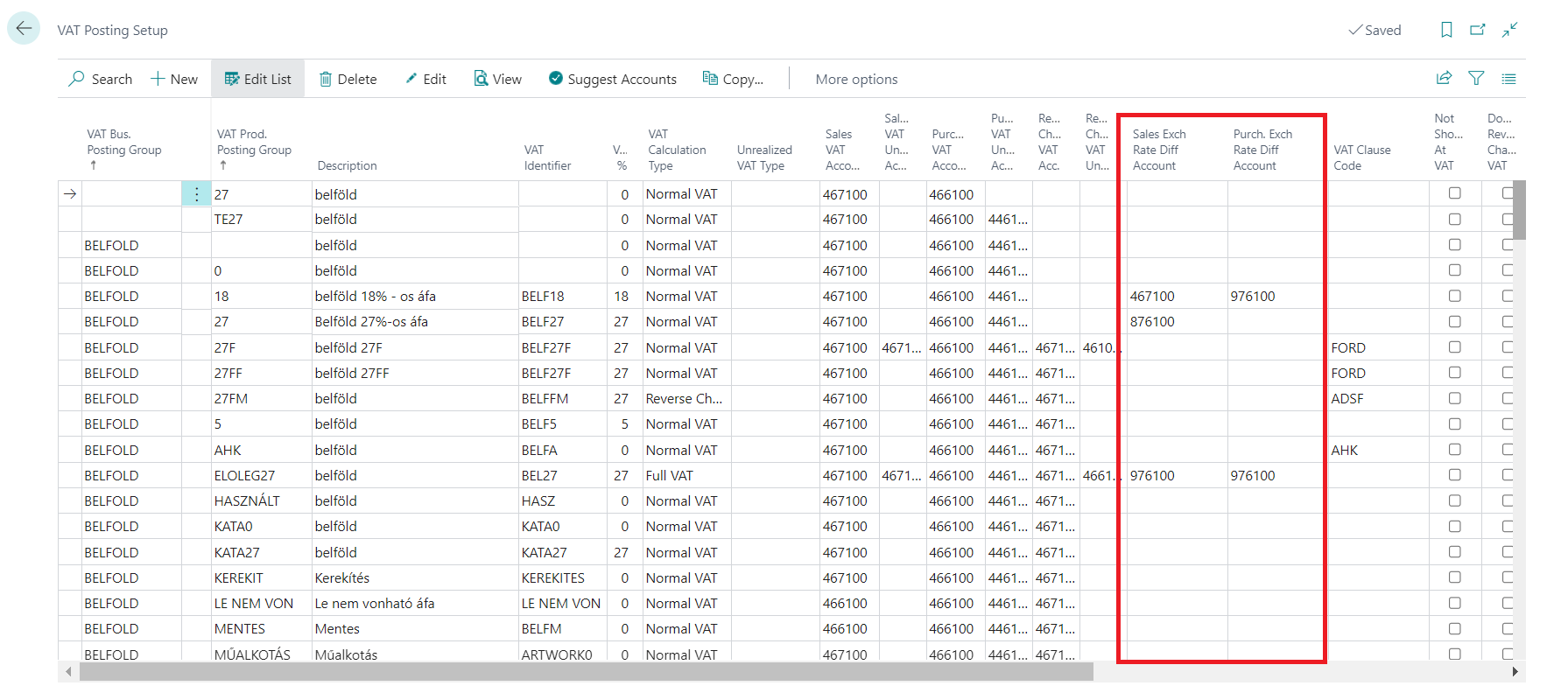Accounting preview of the example, for an invoice of 1000 EUR + VAT: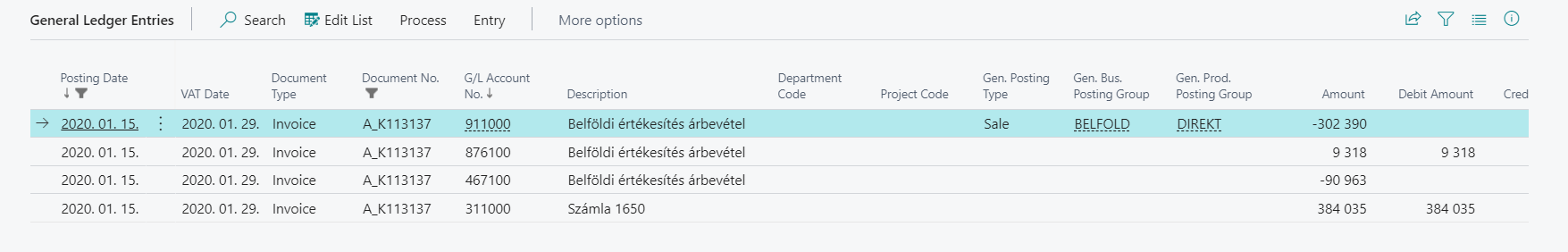Explanation

• 302,39 x 1 270 = 384 035
• 302,39 x 1 000 = 302 390
• 384 035 - 302 390 = 9 318
• 336,9 x 270 = 90 963